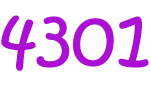# Definition of QuinaryQuinary numbers are made up only of the digits $\{0,1,2,3,4\}$. Each place in a quinary number corresponds to a power of $5$.

Because there are only $5$ different possible digits in a quinary number, we also call them Base $5$ numbers.

For example, the quinary number $4301$ is equal to the decimal number $4 \times 5^3 + 3 \times 5^2 + 0 \times 5^1 + 1 \times 5^0 = 4 \times 125 + 3 \times 25 + 0 + 1 = 576$.

Some other examples of quinary numbers are:

• $44$, which corresponds to the decimal number $24$.
• $100$, which corresponds to the decimal number $25$.
• $104$, which corresponds to the decimal number $29$.

### Description

The aim of this dictionary is to provide definitions to common mathematical terms. Students learn a new math skill every week at school, sometimes just before they start a new skill, if they want to look at what a specific term means, this is where this dictionary will become handy and a go-to guide for a student.

### Audience

Year 1 to Year 12 students

### Learning Objectives

Learn common math terms starting with letter Q

Author: Subject Coach
You must be logged in as Student to ask a Question.